# Background color in ggplot2

Default plot

By default, ggplot2 plots have a gray panel and a white background.

``````library(ggplot2)

# Sample data
set.seed(123)
x <- 1:220
y <- x + rnorm(220, sd = 50)

df <- data.frame(x = x, y = y)

# Plot
ggplot(data = df, aes(x = x, y = y)) +
geom_point()``````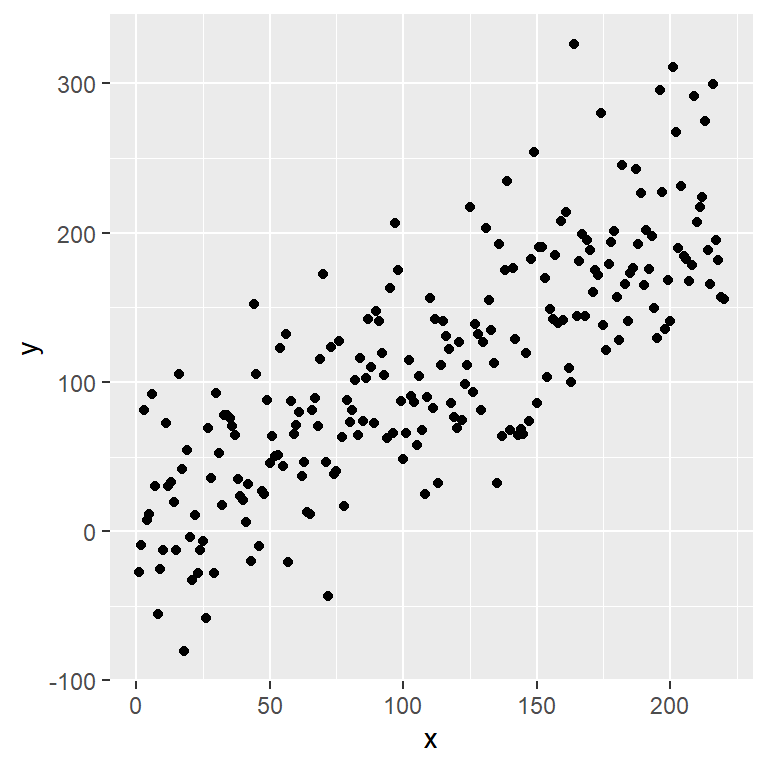## Panel background color

You can change the panel background color setting an `element_rect` in the `panel.background` component of the `theme` function as follows.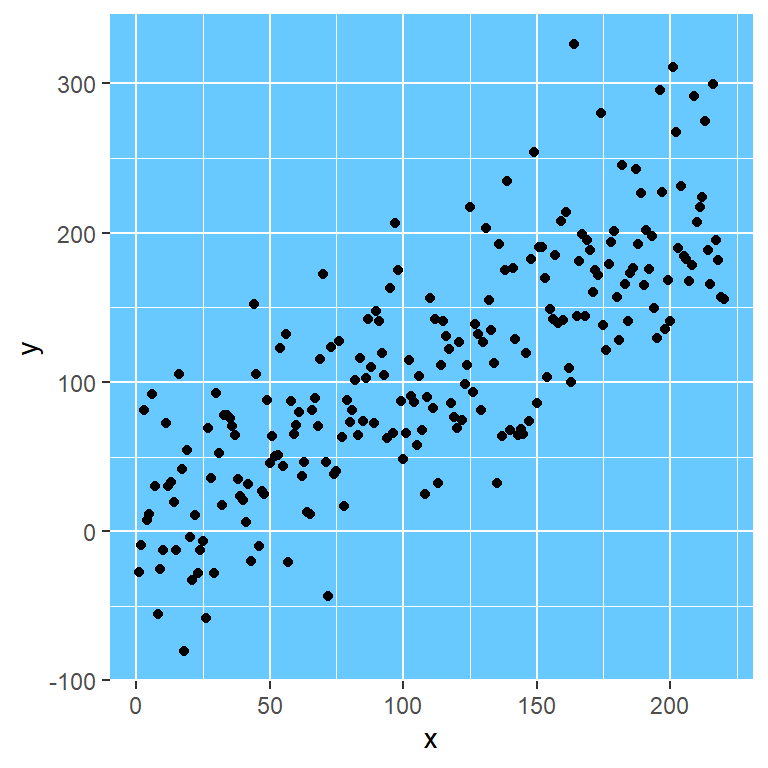``````library(ggplot2)

ggplot(data = mtcars, aes(x = mpg, y = cyl))+
geom_point() +
theme(panel.background = element_rect(fill = "#67c9ff"))``````

## Panel border color

Option 1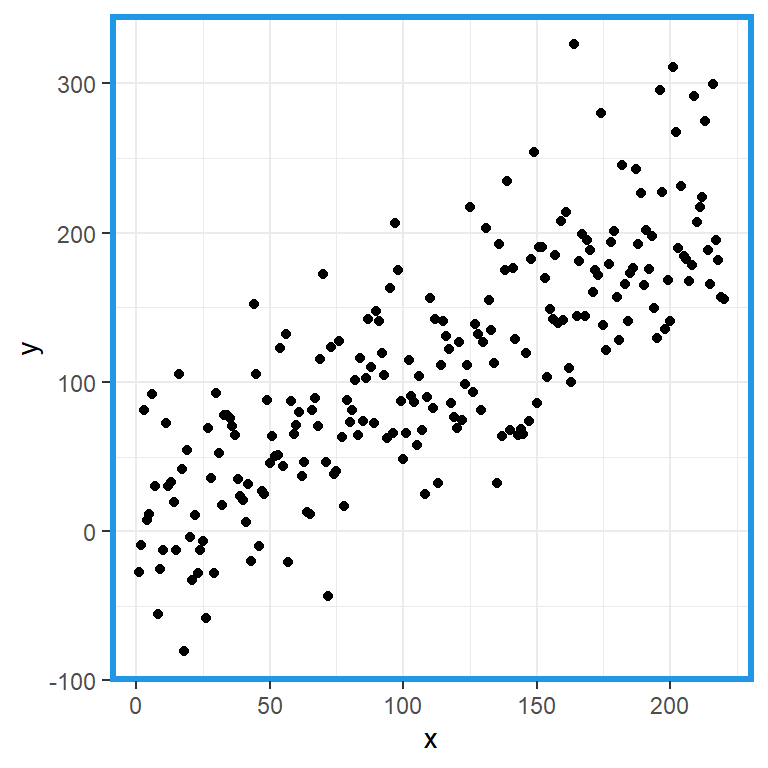The `panel.border` component of the `theme` function controls the color and width of the border of the panel with the `color` and `size` arguments. However, you will need to set `fill = "transparent"` to avoid hiding the data.

``````library(ggplot2)

ggplot(data = df, aes(x = x, y = y)) +
geom_point() +
theme_bw() +
theme(panel.border = element_rect(fill = "transparent", # Needed to add the border
color = 4,            # Color of the border
size = 2))            # Border width``````

Option 2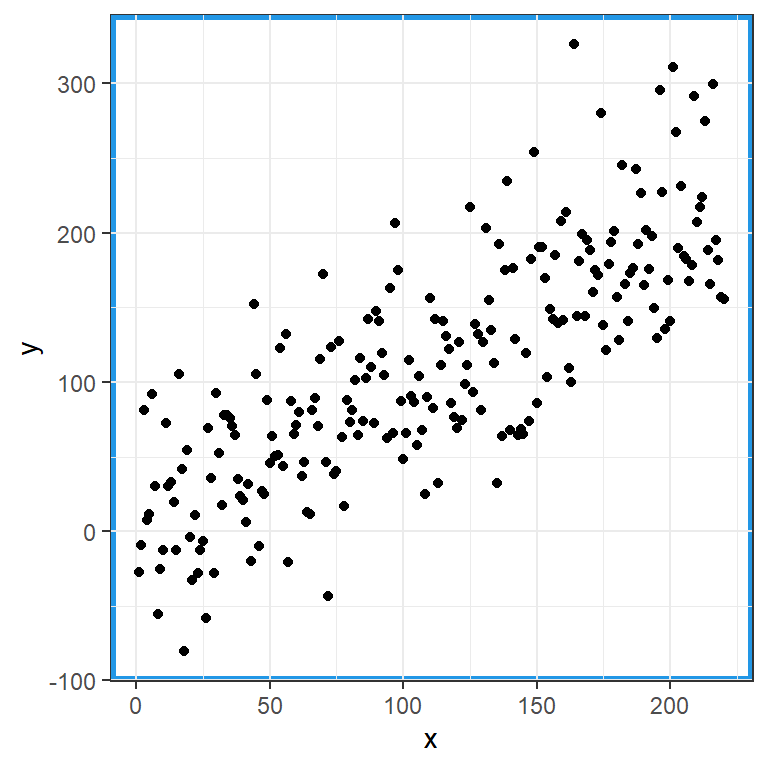You can also set a `element_rect` for the `panel.background` component and modify the border color with the `color` argument. However, this is not the recommended workflow, as it doesn’t override the current border. You can check this with `theme_bw` (Note that the black border is behind the blue border).

``````library(ggplot2)

ggplot(data = df, aes(x = x, y = y)) +
geom_point() +
theme_bw() +
theme(panel.background = element_rect(color = 4, # Color of the border
size = 2)) # Border width``````

## Plot background color

The `plot.background` component of the `theme` function allows modifying the background color of the figure. Set the color inside the `fill` argument of an `element_rect`.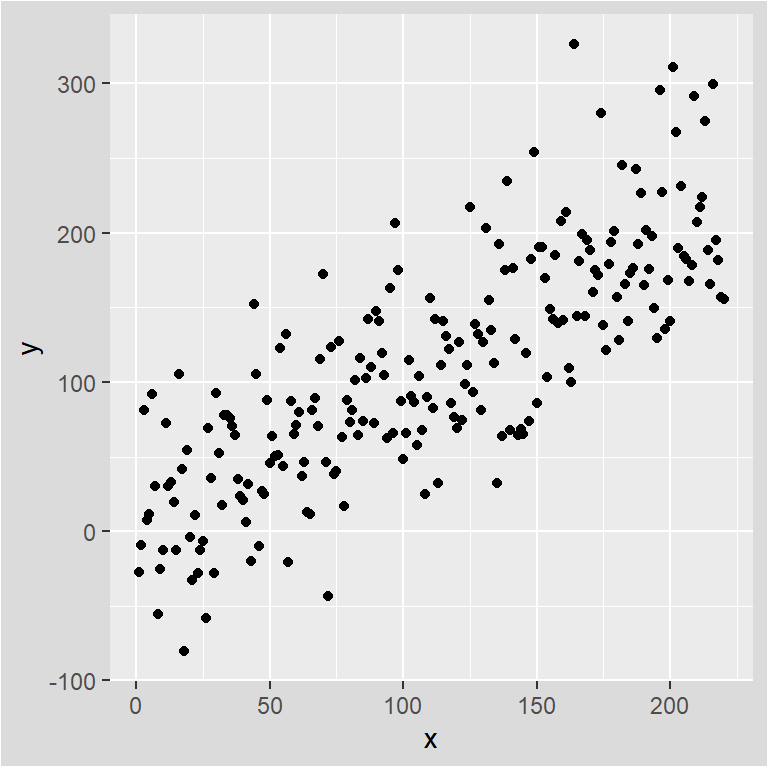``````library(ggplot2)

ggplot(data = mtcars, aes(x = mpg, y = cyl))+
geom_point() +
theme(plot.background = element_rect(fill = "gray86")) # Background color of the plot``````

## Plot border color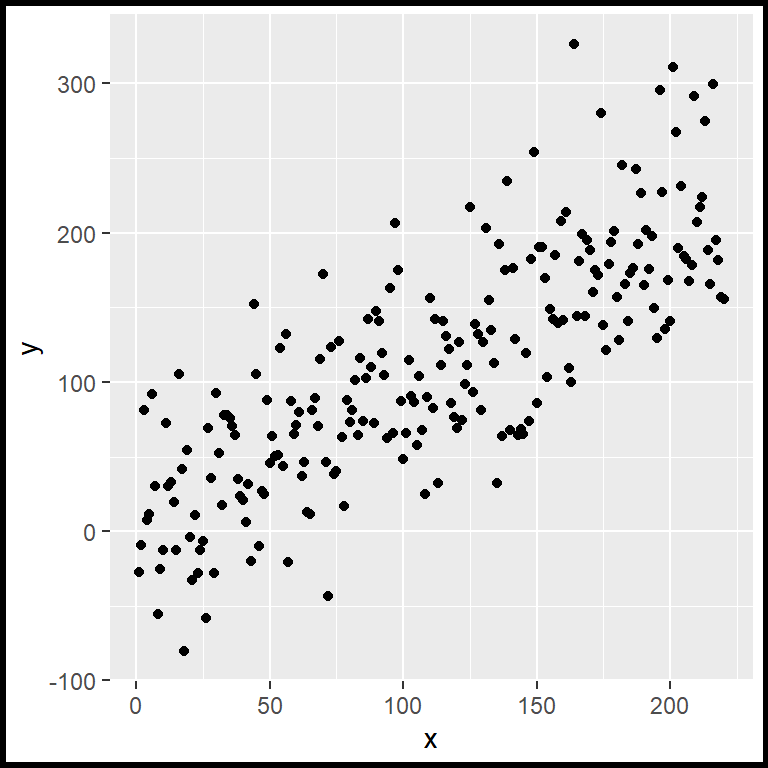You can also set a border color for the whole figure. Just pass an `element_rect` to the `plot.background` component of the `theme` function and modify the color and the width of the border with the arguments `color` and `size`, respectively.

``````library(ggplot2)

ggplot(data = df, aes(x = x, y = y)) +
geom_point() +
theme(plot.background = element_rect(color = "black", # Border color
size = 2))       # Border width``````

## Changing the colors with themes

It is worth mentioning that there are lots of ggplot themes available that provide different background colors.

In this example we are setting the `theme_dark`, which is one of the in-built ggplot2 themes.

``````library(ggplot2)

ggplot(data = df, aes(x = x, y = y)) +
geom_point() +
theme_dark()``````

Note that the default theme is `theme_grey`.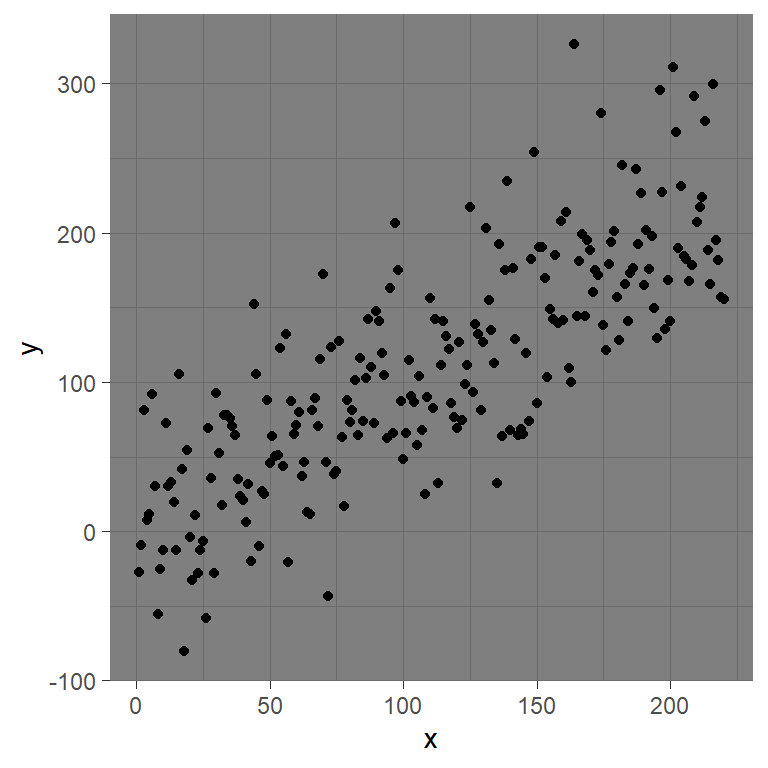## Color picker

Use the color pickers to change the panel color (left) and the plot color (right), or to generate random colors pressing the blue button. Then you can copy the colors and use them in your plots.